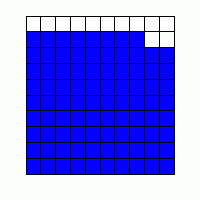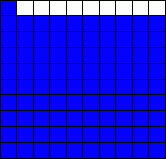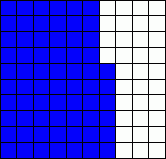MathScore EduFighter is one of the best math games on the Internet today. You can start playing for free!

## Counting Squares - Sample Math Practice Problems

The math problems below can be generated by MathScore.com, a math practice program for schools and individual families. References to complexity and mode refer to the overall difficulty of the problems as they appear in the main program. In the main program, all problems are automatically graded and the difficulty adapts dynamically based on performance. Answers to these sample questions appear at the bottom of the page. This page does not grade your responses.

See some of our other supported math practice problems.

### Complexity=1, Mode=10square

Count the number of shaded squares.

 1# shaded squares: 2# shaded squares:

### Complexity=1, Mode=100grid

Count the number of shaded squares or show how many by counting out the given number. Click when you have the right number of squares.

 1.   18 2.# shaded squares:

### Complexity=1, Mode=beyond100

Count the number of shaded squares.

1.2.### Complexity=1, Mode=10square

Count the number of shaded squares.

12### Complexity=1, Mode=100grid

Count the number of shaded squares or show how many by counting out the given number. Click when you have the right number of squares.

118
2### Complexity=1, Mode=beyond100

Count the number of shaded squares.

1